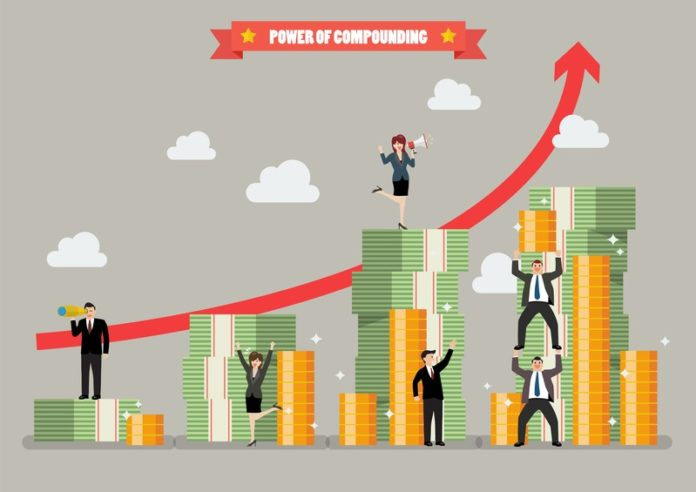# What is Compounding? How to Benefit From It?

0
599Compound return is earned when the interest earned in one period is added back to the principal amount to generate a new principal on which interest is computed for the next period. As a result, interest is reinvested in the asset so that interest is earned on interest.

This picture below compares the value of a rupee when it grows at a simple interest of 10%, as against a compounded annual rate of 10%.At the end of the first year, the rupee would have grown to Rs.1.01, which forms the new principal for the second year if compound interest is calculated, and so on for each following year. Note that with time, the difference between the two options grows, as the power of compound interest kicks in.

The formula for compounding is
FV = PV (1+r)^n
Where
FV= Future Value PV= Present Value r = rate of return for each compounding period n = number of compounding periods

Note that the rate of return for each compounding period has to be adjusted for the frequency of compounding. For example, if an investment pays 8% interest p.a compounded quarterly, then the applicable rate of return for each compounding period is 8%/4, or 2%. The number of compounding periods (n) refers to the periodicity with which interest is paid on the investment during the year. For example, the Post Office Monthly Income Scheme (MIS) pays interest every month, while the Senior Citizens Scheme pays every quarter. The greater the frequency of compounding, the more often interest is paid on interest, and the greater are returns earned through compounding.

Consider the following example. Krishna invests Rs.5 lakhs in a bank deposit that pays 8% interest compounded annually. What is the interest he earns from the investment if
1. The interest is used to pay the college fees of his daughter
2. The cumulative option is chosen and the interest is paid at maturity
3. If the interest is instead compounded quarterly and he chooses the cumulative option

Under Scenario 1
The interest income earned is: Rs.5 lakhs x 8% x 5=Rs.200000 There is no compounding benefit since the interest is taken out and used and not re-invested.

Under scenario 2
The maturity value will be= 500000 x (1+8%)^5 = Rs.734664 Interest income earned over 5 years = Rs.734664- Rs.500000= Rs.23664
The interest income is higher because the interest earned each year is re-invested and earns interest too. This is the compounding benefit.

Under scenario 3
The maturity value will be= 500000 x (1+(8%/4)^20) = 500000 x (1+2%)^20= Rs.742974 Interest income earned over 5 years = Rs.743974- Rs.500000= Rs.242974 The interest income is higher than scenario 2 because the frequency of compounding is higher. The interest is paid each quarter and this earns interest for the remaining period.

To understand more about compound interest, consider the example of Mr. and Mrs. Mony, both aged 30, who are making their respective retirement plans.
Mr Mony plans to invest Rs. 100,000 every year starting from age 45 for 15 years (i.e. he will withdraw the money at the age of 60) and he is expecting a return of about 12%.
Mrs. Mony invests Rs. 100,000 every year starting from age 30 for 30 years (i.e. she will withdraw the money at the age of 60) with a similar return expectation.
Note that both are planning to invest the same amount and expect the same rate of return, but Mrs Mony plans to keep her funds invested for double the time (30 years versus 15 years). The retirement corpus of both can be calculated as follows.

In Plan 1, Mrs Mony’s corpus has grown by more than 6.5 times than of Mr. Mony, due to the longer compounding period. In fact, even if she reduces her annual investment to one-third of the original, say Rs.30,000, her corpus is double that of Mr Mony (Plan 2).
For Mr. Mony to grow his corpus at the same compound rate, given his shorter investment horizon, he needs to double his investment (Plan 3). The power of compounding allows Mrs Mony’s corpus to grow more rapidly.